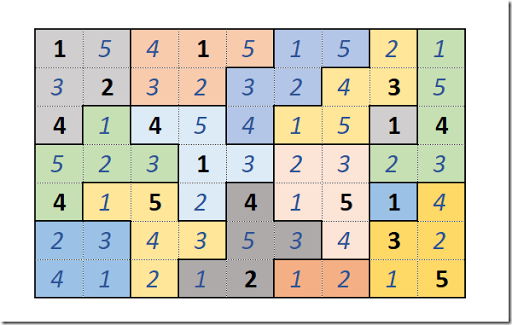## Thursday, November 2, 2017

### Suguru

Suguru is another popular, Sudoku-like puzzle originating from Japan.

We need to fill every block with unique numbers $$1,2,..,n_b$$ where $$n_b$$ is the number of cells in a block. (This implies a singleton block – a block with just one cell – has to contain the value 1. In the puzzle above, the two singleton blocks are already filled in. I am not sure why.) The constraint is that neighboring cells can not contain the same number. Here a neighbor includes the cell diagonally touching (i.e., an interior cell has 8 neighbors).

To solve this as a MIP model, as usual for these type of puzzles (see ), we can define our binary decision variable as:

 $x_{i,j,k}=\begin{cases}1 &\text{if cell $$(i,j)$$ has value k}\\0 & \text{otherwise}\end{cases}$

Here $$k$$ is running from $$1,\dots,n_b$$, i.e. it depends on the block $$b$$ containing cell $$(i,j)$$ . Some of these values can be fixed using the initially given values:

 $x_{i,j,k} = 1 \>\text{if V^0_{i,j}=k}$

where $$V^0$$ are the provided initial values.

Of course only one value can be selected in a cell:

 $\sum_{k=1}^{n_b} x_{i,j,k} = 1\>\>\forall i,j$

In the following we assume we have a map $$B_{b,i,j}$$ which is true if cell $$(i,j)$$ is in block $$b$$. The uniqueness within a block is not very difficult to establish:

 \sum_{i,j|B_{b,i,j}} x_{i,j,k} = 1 \>\>\>\begin{align}&\forall b\\ &\forall k\le n_b\end{align}

Again, only applicable $$k$$'s are to be considered.

Finally, we need to make sure neighboring cells do not have the same value as cell $$(i,j)$$. One way to handle this, is to have a large number of pairwise constraints:

 x_{i,j,k}+x_{i’,j’,k}\le 1\>\>\> \begin{align}&\forall (i,j),(i’,j’) | N_{i,j,i’,j’}\\ &\forall k \le \min\{n_b,n_{b’}\}\end{align}

Here $$N_{i,j,i',j'}$$ indicates whether cells $$(i,j)$$ and $$(i',j')$$ are neighbors. We can restrict this to neighbors that are in different blocks only (we already compared values in the same block). In addition we can cut the number of these constraints in half by observing that if we compared cells $$(i,j)$$ and $$(i',j')$$ we do not have to check $$(i’,j’)$$ and $$(i,j)$$. (See  for a similar symmetry issue.) Also we can restrict $$k$$ to the smallest value $$\min\{n_b,n_{b’}\}$$ where $$(i,j$$) is in $$b$$ and $$(i’,j’)$$ is in $$b’$$. This constraint needs some attention if you want to prevent generating unnecessary pairs.

If you would be generous and ignore all these points you would generate $$\mathit{NumNeigbors} \times \max n_b = 2375$$ of these pairwise equations. Being stingy, we can reduce this to $$503$$ equations.

To display a solution we can calculate:

 $v_{i,j} = \sum_k k \cdot x_{i,j,k}$

We can do this outside the model using the solution values of $$x$$, or we can add a reporting equation to the model (this is sometimes called an “accounting row”) and let the solver calculate the $$v$$ values for us.

There is no objective: we just want to find a feasible solution.

A good solver will say something like:

MIP Presolve eliminated 630 rows and 298 columns.
All rows and columns eliminated.

I.e. the presolve completely solves the model and we don’t have to do any branching.

The solution looks like:The solution is unique. We can get some hard evidence of that by taking this solution $$s_{i,j,k}=x^*_{i,j,k}$$ and adding the constraint:

 $\sum_{i,j,k} s_{i,j,k} x_{i,j,k} \le n-1$

where $$n$$ is the number of cells. If we resolve the problem, the model is declared infeasible. This confirms there is only one, unique solution.

##### Conclusion

Even though this initially looked like a simple problem that can be easily modeled as a MIP problem, there are some interesting wrinkles. This modeling approach is very different from writing an algorithm .

##### References
1. Solving Suguru (Tectonic) puzzles, http://community.wolfram.com/groups/-/m/t/1077888. Contains Matlab code to solve the puzzle. The above example was taken from this document.
2. MIP Modeling: from Sudoku to KenKen via Logarithms, http://yetanothermathprogrammingconsultant.blogspot.com/2016/10/mip-modeling-from-sudoku-to-kenken.html
3. No overlapping jobs, http://yetanothermathprogrammingconsultant.blogspot.com/2017/10/no-overlapping-jobs.html
4. Suguru: GAMS/MIP vs Python/Z3, http://yetanothermathprogrammingconsultant.blogspot.com/2017/11/suguru-gamsmip-vs-pythonz3.html. Compare code in GAMS and Python using a MIP and a Z3 formulation. Conclusion: the data manipulation is more complex than the model constraints themselves.